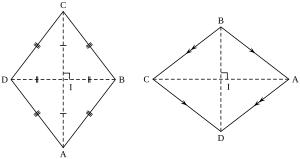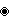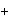# Rhombus facts for kids

Kids Encyclopedia Facts
Quick facts for kids
RhombusTwo rhombi
Edges and vertices 4
Schläfli symbol { } + { }
Coxeter diagramSymmetry group Dihedral (D2), , (*22), order 4
Dual polygon rectangle
Properties convex, isotoxal

A rhombus is a parallelogram with all sides equal in length. A rhombus with all angles equal is called a square.

The word rhombus comes from the Greek word rhombos, meaning "spinning top". A rhombus is sometimes called a diamond, but not all rhombi are diamond shaped.

To find the perimeter of a rhombus, just add up all the sides. Also opposite sides are parallel and opposite angles are equal.

Another interesting thing is that the diagonals (dashed lines in second figure) meet in the middle at a right angle.

## Characterizations

A simple (non-self-intersecting) quadrilateral is a rhombus if and only if it is any one of the following:

• a parallelogram in which a diagonal bisects an interior angle
• a parallelogram in which at least two consecutive sides are equal in length
• a parallelogram in which the diagonals are perpendicular (an orthodiagonal parallelogram)
• a quadrilateral with four sides of equal length (by definition)
• a quadrilateral in which the diagonals are perpendicular and bisect each other
• a quadrilateral in which each diagonal bisects two opposite interior angles
• a quadrilateral ABCD possessing a point P in its plane such that the four triangles ABP, BCP, CDP, and DAP are all congruent
• a quadrilateral ABCD in which the incircles in triangles ABC, BCD, CDA and DAB have a common point

## Basic properties

Every rhombus has two diagonals connecting pairs of opposite vertices, and two pairs of parallel sides. Using congruent triangles, one can prove that the rhombus is symmetric across each of these diagonals. It follows that any rhombus has the following properties:

• Opposite angles of a rhombus have equal measure.
• The two diagonals of a rhombus are perpendicular; that is, a rhombus is an orthodiagonal quadrilateral.
• Its diagonals bisect opposite angles.In Spanish: Rombo para niños